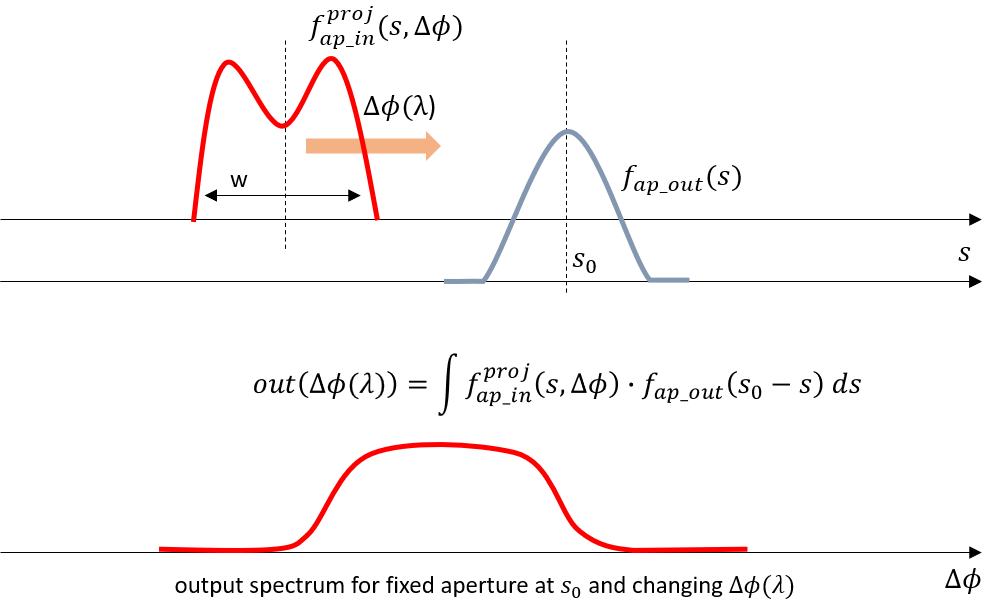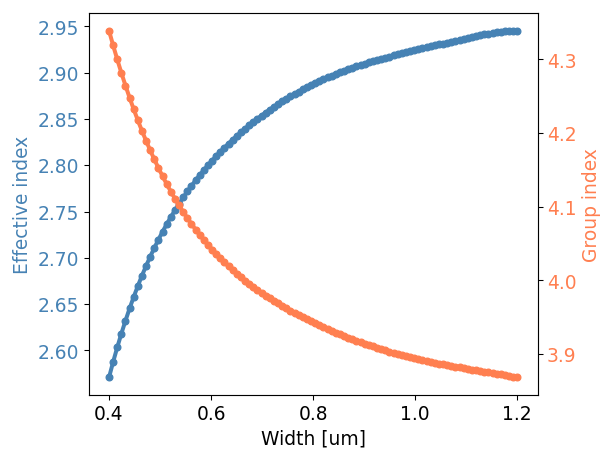# 3.2. AWG generation: Subcomponents¶

In order to design and implement an AWG, we need a few basic aspects and components:

For each of these components, we need to ensure the parameters are matching our technology and application. Once we control these, we can design an AWG and define its input and output star couplers and its waveguide array. The simulation of AWGs by the AWG Designer is performed using a hierarchical model, which builds up a model of the complete AWG by taking the models of its subcomponents, starting from the material models.

## 3.2.1. Materials¶

The material models (real part n and imaginary part k of the refractive index) are used to calculate slab waveguide modes (slab template) and aperture waveguide modes. Therefore, we need to ensure that the correct material model as a function of wavelength is used.

Every IPKISS PDK contains material models. In this tutorial, we are using the SiFab PDK, which contains silicon and silicon dioxide material models. Each optical material has a get_n() method which we can use to check its refractive index as a function of wavelength, as illustrated in the code below.

import si_fab.all as pdk
import ipkiss3.all as i3
import numpy as np
import pylab as plt

TECH = i3.get_technology()

wavelengths = np.linspace(1.25, 1.35, 100)

n_si = [
TECH.MATERIALS.SILICON.get_n(i3.Environment(wavelength=wavelength)).real
for wavelength in wavelengths
]

n_sio2 = [
TECH.MATERIALS.SILICON_OXIDE.get_n(i3.Environment(wavelength=wavelength)).real
for wavelength in wavelengths
]

plt.subplot(211)
plt.plot(wavelengths, n_si, 'b-', linewidth=5)
plt.xlabel('Wavelength [um]')
plt.ylabel('n')
plt.title('Silicon refractive index')
plt.subplot(212)
plt.plot(wavelengths, n_sio2, 'b-', linewidth=5)
plt.xlabel('Wavelength [um]')
plt.ylabel('n')
plt.title(r'SiO2 refractive index')
plt.tight_layout()
plt.show()


Open plot_material.py and run it to visualize the material models in the SiFab PDK. Is this the behaviour you would expect from silicon and silicon dioxide?

File location: luceda-academy/training/topical_training/cwdm_awg/components/plot_material.py

## 3.2.2. Slab template¶

The template si_fab_awg.all.SiSlabTemplate describes the cross-section and modes of the SOI slab waveguide. It is implemented in si_fab, in the si_fab_awg module.

We can visualize its properties to ensure correctness. The cross-section can be obtained from its layout view:

import si_fab.all as pdk
from si_fab_awg.all import SiSlabTemplate
import numpy as np

# Instantiate slab
slab = SiSlabTemplate()

# Visualize cross-section
slab_layout = slab.Layout()
slab_xs = slab_layout.cross_section()
slab_xs.visualize()



The slab waveguide’s modes are obtained automatically by running a CAMFR simulation on the cross-section. Two inputs are essential:

1. The wavelength range within which we calculate the modes. Here, we select the band between 1.25 um and 1.35 um.
2. The material models, verified in the previous step.

The resulting mode effective indices can be plotted and checked easily:

# Calculate and visualize modes
wavelengths = np.linspace(1.25, 1.35, 101)
slab_modes = slab.SlabModesFromCamfr(wavelengths=wavelengths)
slab_modes.visualize(wavelengths=wavelengths)


Open plot_slab.py and run it to visualize the guided modes of the SOI slab waveguide. Is this the behaviour you would expect? Why is the effective index for TM0 lower than the one for TE0?

File location: luceda-academy/training/topical_training/cwdm_awg/components/plot_slab.py

## 3.2.3. Waveguide aperture¶

In order to couple light efficiently into and out of the star coupler with low reflection or scattering, we will use a rib waveguide with partially etched silicon. The si_fab_awg module contains si_fab_awg.all.SiRibAperture which transitions from a single-mode fully etched strip waveguide to a broader (multimode) rib waveguide and finally into the SOI slab waveguide characterized by the si_fab_awg.all.SiSlabTemplate.

It is important to explicitly define which slab template the aperture opens into, and in particular the wavelength range of the slab’s model:

import si_fab.all as pdk
from si_fab_awg.all import SiSlabTemplate, SiRibAperture
import ipkiss3.all as i3
import numpy as np

wavelengths = np.linspace(1.25, 1.35, 100)
center_wavelength = 1.3

slab_tmpl = SiSlabTemplate()
slab_tmpl.SlabModesFromCamfr(wavelengths=wavelengths)

aperture = SiRibAperture(
slab_template=slab_tmpl,
taper_length=30.0,
aperture_core_width=2.0,
wire_width=0.4,
)

# Layout
print("Instantiating aperture layout...\n")
aperture_lay = aperture.Layout()
aperture_lay.visualize(annotate=True)
aperture_lay.visualize_2d(process_flow=i3.TECH.VFABRICATION.PROCESS_FLOW_FEOL)



The slab template is used by the aperture in two ways:

1. The field profile at the aperture opening (the output of the transition) is calculated as an expansion of the slab modes
2. The far field is calculated using the properties of the slab modes

First, let’s check the output field at the aperture into the slab. In this case, it is calculated automatically using CAMFR, similarly to Multi-mode interferometer (MMI).

# Field model
fieldmodel = aperture.FieldModelFromCamfr()
env = i3.Environment(wavelength=center_wavelength)

# Field profile at the aperture
print("Simulating field profile at aperture...\n")
fields = fieldmodel.get_fields(mode=0, environment=env)
fields.visualize()



We can also check a top-down view of the field propagating through the whole aperture structure:

# Field propagated from input to the aperture
print("Simulating aperture field propagation...\n")
fields_2d = fieldmodel.get_aperture_fields2d(mode=0, environment=env)
fields_2d.visualize()



Note that this field profile is not necessarily exactly the same as the ground mode profile of the transition’s output! Instead, it is the field profile expanded into the slab modes, after having taken the overlap integral with those slab modes.

Finally, we can have a look at the far field of the aperture and its $$1/e^2$$ divergence angle:

# Far field of the aperture
print("Simulating far field of the aperture...\n")
fields_ff = fieldmodel.get_far_field(mode=0, environment=env)
divergence_angle = fields_ff.divergence_angle(slab_mode='TE0', method='e_sq')
print(divergence_angle)
fields_ff.visualize()


Run the following file:

luceda-academy/training/topical_training/cwdm_awg/components/plot_aperture.py

• Change taper_length. What is the influence on the transmission? Can the taper be made shorter?
• Change aperture_core_width What is the influence on the transmission?

## 3.2.4. Multimode interference aperture¶

A well-known technique to achieve a broad transmission band around each channel wavelength, is to use a multimode interference (MMI) aperture at the input side while keeping the regular aperture at the output side.

The channel spectral response is a convolution between the output’s field profile with the image of the input field on the output focal plane (multiplied by the array factor of the phased array). Hence, if we design the MMI such that the field launched into the input star coupler has two lobes with a lower field strength in the center, this convolution will yield a more flat-top behavior. This will come at the expense of somewhat higher insertion loss.Fig. 3.1 Flat-top behavior with an MMI input: the convolution of the projection of the input field (output of the MMI) with the output aperture’s field along the output curve yields a flat-top spectrum.

The SiFab PDK contains a predefined si_fab_awg.all.SiRibMMIAperture. Like for the regular aperture, we can instantiate it and visualize its layout top-down. Then we can plot the output field profile, the 2D top-down view of the fields and the far-field.

import si_fab.all as pdk
from si_fab_awg.all import SiSlabTemplate, SiRibMMIAperture
import ipkiss3.all as i3
import numpy as np

wavelengths = np.linspace(1.25, 1.35, 100)
center_wavelength = 1.3

slab_tmpl = SiSlabTemplate()
slab_tmpl.SlabModesFromCamfr(wavelengths=wavelengths)

aperture = SiRibMMIAperture(slab_template=slab_tmpl, mmi_length=9.0, wire_width=0.4)

# Layout
print("Instantiating aperture layout...\n")
aperture_lay = aperture.Layout()
aperture_lay.visualize(annotate=True)
aperture_lay.visualize_2d(process_flow=i3.TECH.VFABRICATION.PROCESS_FLOW_FEOL)

# Field model
fieldmodel = aperture.FieldModelFromCamfr()
env = i3.Environment(wavelength=center_wavelength)

# Field profile at the aperture
print("Simulating field profile at aperture...\n")
fields = fieldmodel.get_fields(mode=0, environment=env)
fields.visualize()

# Field propagated from input to the aperture
print("Simulating aperture field propagation...\n")
fields_2d = fieldmodel.get_aperture_fields2d(mode=0, environment=env)
fields_2d.visualize()

# Far field of the aperture
print("Simulating far field of the aperture...\n")
fields_ff = fieldmodel.get_far_field(mode=0, environment=env)
divergence_angle = fields_ff.divergence_angle(slab_mode='TE0', method='e_sq')
print(divergence_angle)
fields_ff.visualize()


With this MMI aperture, the regular aperture and the slab template, we have now reviewed the components that are needed to design the star couplers of the AWG.

Run the following file:

luceda-academy/training/topical_training/cwdm_awg/components/plot_mmi_aperture.py

• Change mmi_length. What is the influence on the transmission?

## 3.2.5. Waveguides¶

Finally, we need a model for the waveguides, so that the waveguide array can be correctly designed, generated and simulated. The waveguide’s wavelength-dependent effective index model will be used to design the length increments between successive waveguides to match the required specifications.

SiFab already comes with a strip waveguide template with an appropriate model. Here, we visualize its effective index and group index at 1.3 um wavelength:

import si_fab.all as pdk
import ipkiss3.all as i3
import numpy as np
import pylab as plt

center_env = i3.Environment(wavelength=1.3)
widths = np.linspace(0.4, 1.2, 100)
neffs = []
ngs = []

for w in widths:
tmpl = pdk.SiWireWaveguideTemplate()
tmpl.Layout(core_width=w)
tmpl_cm = tmpl.CircuitModel()
neffs.append(tmpl_cm.get_n_eff(center_env))
ngs.append(tmpl_cm.get_n_g(center_env))

fig, ax1 = plt.subplots()

ax1.plot(widths, neffs, 'bo-', markersize=5, linewidth=3, color='steelblue')
ax1.set_xlabel('Width [um]', fontsize=14)
ax1.set_ylabel('Effective index', color='steelblue', fontsize=14)
ax1.tick_params(axis='y', labelcolor='steelblue', labelsize=14)
ax1.tick_params(axis='x', labelcolor='black', labelsize=14)

ax2 = ax1.twinx()
ax2.plot(widths, ngs, 'ro-', markersize=5, linewidth=3, color='coral')
ax2.set_ylabel('Group index', color='coral', fontsize=14)
ax2.tick_params(axis='y', labelcolor='coral', labelsize=14)

fig.tight_layout()
plt.show()luceda-academy/training/topical_training/cwdm_awg/components/plot_wg_model